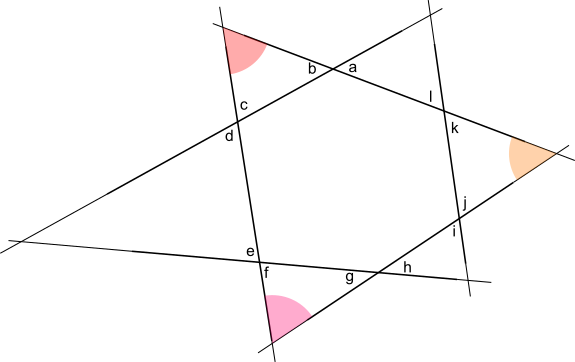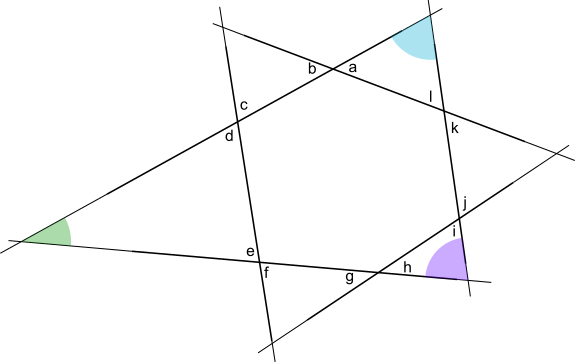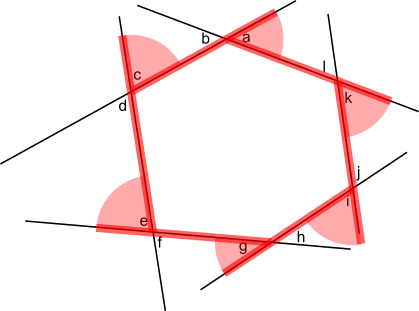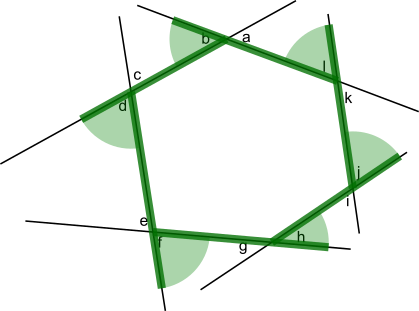You may also likeHallway Borders

What are the possible dimensions of a rectangular hallway if the number of tiles around the perimeter is exactly half the total number of tiles?Square Pegs

Which is a better fit, a square peg in a round hole or a round peg in a square hole?Boxed In

A box has faces with areas 3, 12 and 25 square centimetres. What is the volume of the box?

Age 11 to 14 ShortChallenge Level
Using interior angles of triangles
In the diagram below, all of the lines have been extended so that they cross.

b, c and the red angle form a triangle so add up to 180$^\text o$.
The same is true of f, g and the pink angle and of j, k and the orange angle.

So adding up all of the angles in all 3 triangles gives
b + c + red angle + f + g + pink angle + j + k + orange angle = 180$^\text o$ + 180$^\text o$ + 180$^\text o$

But the red angle, the pink angle and the orange angle are also the angles of a large triangle. So they also add up to 180$^\text o$.

That leaves b + c + f + g + j + k = 180$^\text o$ + 180$^\text o$ = 360$^\text o$.Using the diagram below in the same way, l + a + d + e + h + i is also equal to 360$^\text o$.So the sum of all of the angles denoted by letters is 360$^\text o$ + 360$^\text o$ = 720$^\text o$.

Using the exterior angles of hexagonsImagine walking clockwise around the hexagon shown in red in the diagram on the right. The angles a, k, i, g, e and c are the angles that you would need to turn through to be facing in the direction of the next side.

By the time you got back to where you started from, you would be facing the same way you were facing when you started. So you would have turned through 360$^\text o$.

So a + k + i + g + e + c = 360$^\text o$.Now imagine walking anticlockwise around the hexagon, as shown in green.

Similarly,
b + d + f + h + j + l = 360$^\text o$.

So the sum of all of the angles denoted by letters is
360$^\text o$ + 360$^\text o$ = 720$^\text o$.
You can find more short problems, arranged by curriculum topic, in our short problems collection.